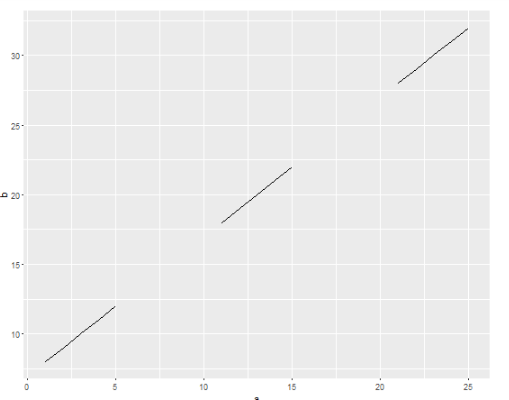# How to add line plots to ggplot2 in a loop?

1.4K    Asked by SuhaniRodrigues in Data Science , Asked on May 1, 2020

We can define the data that is used in a ggplot layer with the data argument:

pl = pl + geom_line(data = df, aes(x = x, y = y), linetype = lt, colour = co)

If data is not specified it will assume that the layer is actually the same each time so it will only show the most recent one. We can test it with following code:

library(ggplot2)

dat <- list(

data.frame(a = 1:5, b = 8:12),

data.frame(a = 11:15, b = 18:22),

data.frame(a = 21:25, b = 28:32)

)

p <- ggplot()

i <- 1

while(i <= length(dat)) {

df <- dat[[i]]

p <- p + geom_line(data = df, aes(a, b))

i <- i + 1

}

P

It will give result something like this